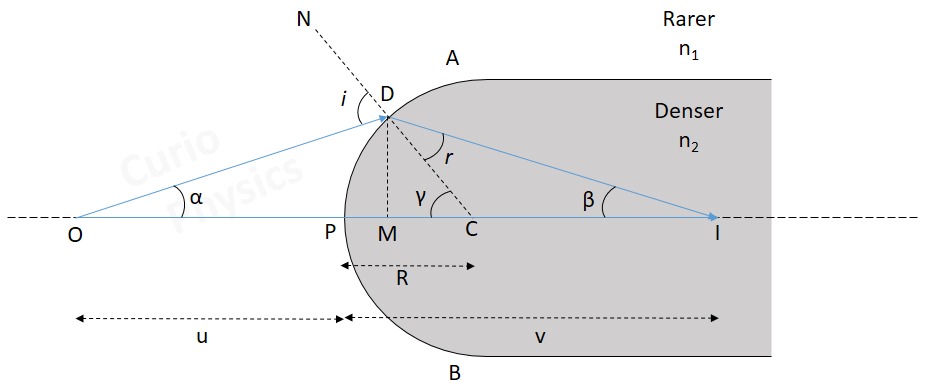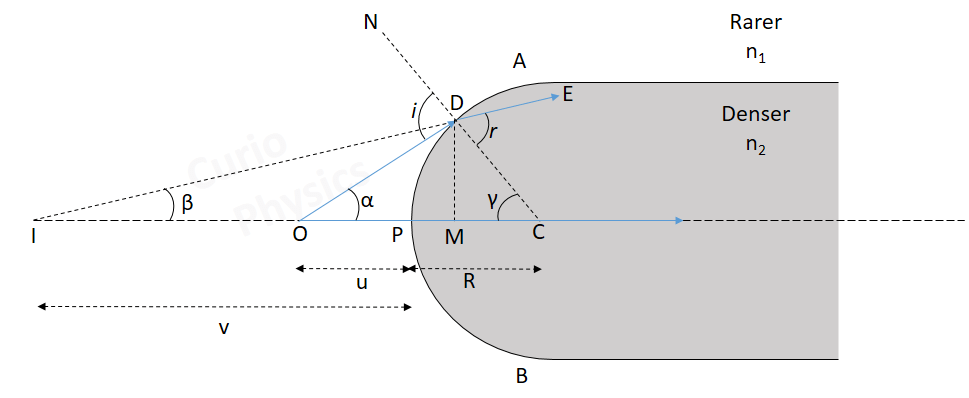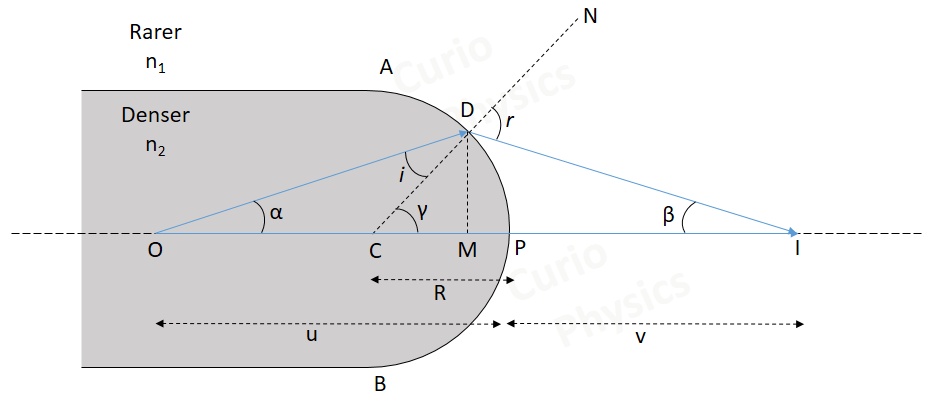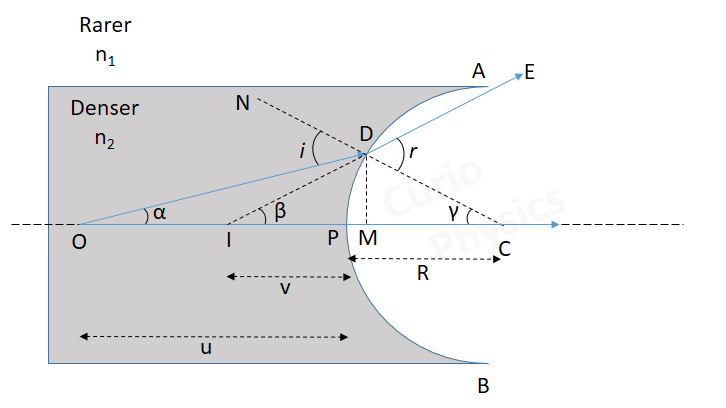# Spherical Refracting Surface

##### Spherical Refracting Surface :-

A refracting surface which forms a part of a sphere of transparent refracting material is called a spherical reflecting surface. These are of two types:

(i) Convex spherical reflecting surface which is convex towards rarer medium side and

(ii) Concave spherical reflecting surface which is concave towards the rarer medium size.Pole(P) :- The centre of the spherical reflecting surface is called its pole.

Centre of curvature(C) :-  Centre of curvature of the spherical reflecting surface is the centre of the sphere of which this surface is a part.

Radius of curvature(R):- The radius of the sphere of which spherical reflecting surface is a part, is called its radius of curvature. In the above figure radius of curvature, PC = R.

Principal axis:- The line passing through the pole and the center of curvature of spherical reflecting surface is called it’s principal axis.

##### New Cartesian sign convention for spherical reflecting surface

(1) All the distances are measured from the pole of the spherical reflecting surface.
(2) The distances measured in the direction of incident light rays are taken as positive and the distance is measured in a direction opposite to the direction of incident light rays are taken as negative.

Note :- When an object faces a convex spherical reflecting surface, radius of curvature R is taken as positive and when an object faces a concave spherical reflecting surface, radius of curvature R is taken as negative.

##### assumptions while dealing with spherical reflecting surface

(1) The object is assumed as a point object lying on the principal axis of the spherical reflecting surface.

(2) The aperture of the spherical reflecting surface is assumed to be small.

(3) The incident and reflected rays make small angles with the principal axis of the spherical reflecting surface so that sin i ≈ i and sin r ≈ r.

##### Refraction of light at spherical refracting surface and image formation

The different possibilities of refraction of light while dealing with spherical refracting surfaces is explained below :-##### (1) Refraction of light from rarer to denser medium at a convex spherical reflecting surface

Here two cases arise. The image formed may be real or virtual.

(a) Real Image :-

Let us consider a point object O is lying on the principal axis of a convex spherical reflecting surface AB.Here we have considered two light rays starting from O. One light ray is incident on spherical reflecting surface at point D(angle of incidence = i) and is refracted along DI(angle of refraction = r).  Another light ray is incident normally on the surface and passes undeviated along the straight line OI.

Both of these light rays actually meet at point I, which is the real image of point O.

Here DM ⊥ principal axis

As we know that external angle of a triangle is equal to the sum of internal opposite angles, therefore, in ΔODC

i = α + γ     …..(1)

Similarly, in ΔIDC

γ = r + β

r = γ – β     …..(2)

Applying Snell’s Law at point D,

n1 sin i = n2 sin r

But since the angles are small, hence

n1 i = n2 r     …..(3)

Using (1) and (2) in (3), we get,

n1 (α + γ) = n2 (γ – β)     …..(4)

While dealing with spherical refracting surface we assume that aperture AB is small. So angles α,β and γ are small. Hence$\displaystyle \tan \alpha \cong \alpha =\frac{DM}{OM}$$\displaystyle \tan \beta \cong \beta =\frac{DM}{IM}$$\displaystyle \tan \gamma \cong \gamma =\frac{DM}{CM}$

Again, as the aperture is small, so M is close to P. Therefore,

OM ≅ OP,  IM ≅ IP and CM ≅ CP$\displaystyle \tan \alpha \cong \alpha =\frac{DM}{OP}$$\displaystyle \tan \beta \cong \beta =\frac{DM}{IP}$$\displaystyle \tan \gamma \cong \gamma =\frac{DM}{CP}$

Let us put the values of α,β and γ in equation (4),$\displaystyle {{n}_{1}}\left( \frac{DM}{OP}+\frac{DM}{CP} \right)={{n}_{2}}\left( \frac{DM}{CP}-\frac{DM}{IP} \right)$$\displaystyle \Rightarrow {{n}_{1}}\left( \frac{1}{OP}+\frac{1}{CP} \right)={{n}_{2}}\left( \frac{1}{CP}-\frac{1}{IP} \right)$$\displaystyle \therefore \left( \frac{{{n}_{1}}}{OP}+\frac{{{n}_{2}}}{IP} \right)=\left( \frac{{{n}_{2}}}{CP}-\frac{{{n}_{1}}}{CP} \right)$

Using new Cartesian sign convention, i.e.,

OP = -u, IP = +v, CP = +R$\displaystyle \frac{{{n}_{2}}}{v}-\frac{{{n}_{1}}}{u}=\frac{{{n}_{2}}-{{n}_{1}}}{R}$     …..(5)

(b) Virtual Image :-

If the point object O lies very close to the pole of the spherical refracting surface, then a virtual image is formed as shown in figure below :-Here the two light rays emerging from point object O, one refracted at point D and moving along DE and the other OC, do not meet actually at any point but appears to come from from point I. Thus I is the virtual image of point object O.

Here DM ⊥ principal axis

In ΔODC,

i = α + γ    …..(6)

Similarly, in ΔIDC

r = γ + β    …..(7)

Applying Snell’s Law at point D,

n1 sin i = n2 sin r

But since the angles are small, hence

n1 i = n2 r     …..(8)

Using (6) and (7) in (8), we get,

n1 (α + γ) = n2 (γ + β)     …..(9)

As the aperture AB is small. So angles α,β and γ are small. Hence$\displaystyle \tan \alpha \cong \alpha =\frac{DM}{OM}$$\displaystyle \tan \beta \cong \beta =\frac{DM}{IM}$$\displaystyle \tan \gamma \cong \gamma =\frac{DM}{CM}$

Again, as the aperture is small, so M is close to P. Therefore,

OM ≅ OP,  IM ≅ IP and CM ≅ CP$\displaystyle \tan \alpha \cong \alpha =\frac{DM}{OP}$$\displaystyle \tan \beta \cong \beta =\frac{DM}{IP}$$\displaystyle \tan \gamma \cong \gamma =\frac{DM}{CP}$

Let us put the values of α,β and γ in equation (9),$\displaystyle {{n}_{1}}\left( \frac{DM}{OP}+\frac{DM}{CP} \right)={{n}_{2}}\left( \frac{DM}{CP}+\frac{DM}{IP} \right)$$\displaystyle \Rightarrow {{n}_{1}}\left( \frac{1}{OP}+\frac{1}{CP} \right)={{n}_{2}}\left( \frac{1}{CP}+\frac{1}{IP} \right)$$\displaystyle \therefore \frac{{{n}_{1}}}{OP}-\frac{{{n}_{2}}}{IP}=\frac{{{n}_{2}}}{CP}-\frac{{{n}_{1}}}{CP}$

Using new Cartesian sign convention, i.e.,

OP = -u, IP = -v, CP = +R$\displaystyle \frac{{{n}_{1}}}{-u}-\frac{{{n}_{2}}}{-v}=\frac{{{n}_{2}}}{R}-\frac{{{n}_{1}}}{R}$$\displaystyle \Rightarrow \frac{{{n}_{2}}}{v}-\frac{{{n}_{1}}}{u}=\frac{{{n}_{2}}-{{n}_{1}}}{R}$     …..(10)

##### (2) Refraction of light from rarer to denser medium at a concave spherical reflecting surface

In this case, only virtual image is formedLet O be a point object lying on the principal axis of a concave spherical refracting surface of aperture AB. A ray of light OD emerging from O and incident at point D, bends towards the normal CDN and is refracted along DE. Another light ray from point object O falls normally on the surface and passes undeviated along OP.

These two light rays DE and OP do not actually meet at any point but appear to come from point I. Thus I is the virtual image of point object O.

In ΔODC, γ = i + α

i = γ – α     …..(11)

Similarly, in ΔIDC, γ = β + r

⇒ r = γ – β     …..(12)

Applying Snell’s Law at point D,

n1 i = n2 r     …..(13)

(because angles are small)

Using (11) and (12) in (13), we get,

n1 (γ – α) = n2 (γ – β)     …..(14)

As done above….$\displaystyle \tan \alpha \cong \alpha =\frac{DM}{OM}$$\displaystyle \tan \beta \cong \beta =\frac{DM}{IM}$$\displaystyle \tan \gamma \cong \gamma =\frac{DM}{CM}$

Again, as the aperture is small, so M is close to P. Therefore,

OM ≅ OP,  IM ≅ IP and CM ≅ CP$\displaystyle \tan \alpha \cong \alpha =\frac{DM}{OP}$$\displaystyle \tan \beta \cong \beta =\frac{DM}{IP}$$\displaystyle \tan \gamma \cong \gamma =\frac{DM}{CP}$

Let us put the values of α,β and γ in equation (14),$\displaystyle {{n}_{1}}\left( \frac{DM}{CP}-\frac{DM}{OP} \right)={{n}_{2}}\left( \frac{DM}{CP}-\frac{DM}{IP} \right)$$\displaystyle {{n}_{1}}\left( \frac{1}{CP}-\frac{1}{OP} \right)={{n}_{2}}\left( \frac{1}{CP}-\frac{1}{IP} \right)$$\displaystyle \therefore \frac{{{n}_{2}}}{IP}-\frac{{{n}_{1}}}{OP}=\frac{{{n}_{2}}}{CP}-\frac{{{n}_{1}}}{CP}$

Using new Cartesian sign convention, i.e.,

OP = -u, IP = -v, CP = -R$\displaystyle \frac{{{n}_{2}}}{-v}-\frac{{{n}_{1}}}{-u}=\frac{{{n}_{2}}}{-R}-\frac{{{n}_{1}}}{-R}$$\displaystyle \Rightarrow \frac{{{n}_{2}}}{v}-\frac{{{n}_{1}}}{u}=\frac{{{n}_{2}}-{{n}_{1}}}{R}$     …..(15)

[ Note that equations (5), (10) and (15) are same]

##### (3) Refraction of light from denser to rarer medium at spherical reflecting surface

(a) At a convex spherical refracting surface (Image formed is Real) :-Let O be a point object lying on the principal axis of a convex(convex towards the rarer medium) spherical refracting surface of aperture AB.

A ray of light OD emerging from O and incident at point D, bends away from the normal CDN and is refracted along DI. Another light ray from point object O falls normally on the surface and passes undeviated along OPI.

These two light rays DI and PI actually meet at point I, which is the real image of point object O.

Here DM ⊥ principal axis

In ΔODC, γ = α + i

⇒ i = γ – α     …..(16)

Similarly, in ΔIDC,

r = γ + β     …..(17)

Applying Snell’s Law at point D,

n2 i = n1 r     …..(18)

(because angles are small)

Using (16) and (17) in (18), we get,

n2 (γ – α) = n1 (γ + β)     …..(19)

Again$\displaystyle \tan \alpha \cong \alpha =\frac{DM}{OM}$$\displaystyle \tan \beta \cong \beta =\frac{DM}{IM}$$\displaystyle \tan \gamma \cong \gamma =\frac{DM}{CM}$

As the aperture is small, so M is close to P. Therefore,

OM ≅ OP,  IM ≅ IP and CM ≅ CP$\displaystyle \tan \alpha \cong \alpha =\frac{DM}{OP}$$\displaystyle \tan \beta \cong \beta =\frac{DM}{IP}$$\displaystyle \tan \gamma \cong \gamma =\frac{DM}{CP}$

Let us put the values of α,β and γ in equation (19),$\displaystyle {{n}_{2}}\left( \frac{DM}{CP}-\frac{DM}{OP} \right)={{n}_{1}}\left( \frac{DM}{CP}+\frac{DM}{IP} \right)$$\displaystyle {{n}_{2}}\left( \frac{1}{CP}-\frac{1}{OP} \right)={{n}_{1}}\left( \frac{1}{CP}+\frac{1}{IP} \right)$$\displaystyle \therefore -\frac{{{n}_{1}}}{IP}-\frac{{{n}_{2}}}{OP}=\frac{{{n}_{1}}}{CP}-\frac{{{n}_{2}}}{CP}$

Using new Cartesian sign convention, i.e.,

OP = -u, IP = +v, CP = -R$\displaystyle -\frac{{{n}_{1}}}{v}-\frac{{{n}_{2}}}{-u}=\frac{{{n}_{1}}}{-R}-\frac{{{n}_{2}}}{-R}$$\displaystyle \Rightarrow \frac{{{n}_{1}}}{v}-\frac{{{n}_{2}}}{u}=\frac{{{n}_{1}}-{{n}_{2}}}{R}$     …..(20)

(b) At a concave spherical refracting surface (Image formed is Virtual) :-Let O be a point object lying on the principal axis of a concave(concave towards the rarer medium) spherical refracting surface of aperture AB.

A ray of light OD emerging from O and incident at point D, bends away from the normal CDN and is refracted along DE. Another light ray from point object O falls normally on the surface and passes undeviated along OP.

These two light rays DE and OP do not actually meet at any point but appear to come from point I. Thus I is the virtual image of point object O.

In ΔODC,

i = α + γ     …..(21)

Similarly, in ΔIDC,

r = β + γ     …..(22)

Applying Snell’s Law at point D,

n2 i = n1 r     …..(23)

Using (21) and (22) in (23), we get,

n2 (α + γ) = n1 (β + γ)     …..(24)

Now$\displaystyle \tan \alpha \cong \alpha =\frac{DM}{OM}$$\displaystyle \tan \beta \cong \beta =\frac{DM}{IM}$$\displaystyle \tan \gamma \cong \gamma =\frac{DM}{CM}$

As the aperture is small, so M is close to P. Therefore,

OM ≅ OP,  IM ≅ IP and CM ≅ CP$\displaystyle \tan \alpha \cong \alpha =\frac{DM}{OP}$$\displaystyle \tan \beta \cong \beta =\frac{DM}{IP}$$\displaystyle \tan \gamma \cong \gamma =\frac{DM}{CP}$

Let us put the values of α,β and γ in equation (24),$\displaystyle {{n}_{2}}\left( \frac{DM}{OP}+\frac{DM}{CP} \right)={{n}_{1}}\left( \frac{DM}{IP}+\frac{DM}{CP} \right)$$\displaystyle {{n}_{2}}\left( \frac{1}{OP}+\frac{1}{CP} \right)={{n}_{1}}\left( \frac{1}{IP}+\frac{1}{CP} \right)$$\displaystyle \therefore \frac{{{n}_{2}}}{OP}-\frac{{{n}_{1}}}{IP}=\frac{{{n}_{1}}}{CP}-\frac{{{n}_{2}}}{CP}$

Using new Cartesian sign convention, i.e.,

OP = -u, IP = -v, CP = R$\displaystyle \frac{{{n}_{2}}}{-u}-\frac{{{n}_{1}}}{-v}=\frac{{{n}_{1}}}{R}-\frac{{{n}_{2}}}{R}$$\displaystyle \Rightarrow \frac{{{n}_{1}}}{v}-\frac{{{n}_{2}}}{u}=\frac{{{n}_{1}}-{{n}_{2}}}{R}$     …..(25)

[ Note that equations (20) and (25) are same]

Note :- From above discussion we found that…

(1) When the object is placed in rarer medium and real or virtual image is formed on refraction at a convex or concave spherical refracting surface, we proved that$\displaystyle \frac{{{n}_{2}}}{v}-\frac{{{n}_{1}}}{u}=\frac{{{n}_{2}}-{{n}_{1}}}{R}$     …..(26)

and…

(2) When the object is placed in denser medium and real or virtual image is formed on refraction at a convex or concave spherical refracting surface, we found that$\displaystyle \frac{{{n}_{1}}}{v}-\frac{{{n}_{2}}}{u}=\frac{{{n}_{1}}-{{n}_{2}}}{R}$     …..(27)

(3) If we interchange n1 and n2 in equation (26), we get equation (27)

(4) Equations (26) and (27) we can be memorized by a single equation given below :-$\displaystyle \frac{{{n}_{\text{material of refracted ray}}}}{v}-\frac{{{n}_{\text{material of incident ray}}}}{u}=\frac{{{n}_{\text{material of refracted ray}}}-{{n}_{\text{material of incident ray}}}}{R}$

Illustration

An air bubble in glass (n = 1.5) is situated at a distance 3 cm from a spherical surface of diameter 10 cm. At what distance from the surface will the bubble appear if the surface is (a) convex (b) concave.

Solution

In case of refraction from curved surface,$\displaystyle \frac{{{n}_{\text{material of refracted ray}}}}{v}-\frac{{{n}_{\text{material of incident ray}}}}{u}=\frac{{{n}_{\text{material of refracted ray}}}-{{n}_{\text{material of incident ray}}}}{R}$

(a) n material of refracted ray = 1,  n material of incident ray = 1.5,  R = – 5 cm and u = –3 cm$\displaystyle \Rightarrow \frac{1}{v}-\frac{1.5}{(-3)}=\frac{(1-1.5)}{(-5)}$$\displaystyle \Rightarrow v=-2.5cm$

(b) n material of refracted ray = 1, n material of incident ray = 1.5,  R = 5 cm and u = –3 cm$\displaystyle \Rightarrow \frac{1}{v}-\frac{1.5}{(-3)}=\frac{(1-1.5)}{(5)}$$\displaystyle \Rightarrow v=-1.66cm$

Next Topic :- Lens#### Manoj Kumar Verma

Hi, I'm Manoj Kumar Verma, a physics faculty having 7 years of teaching experience. I have done B.Tech (E.E.). I am also a YouTuber and Blogger. This blog is dedicated to help students learn the physics concepts easily.

View all posts

•Rockstar तेजस्वी says:
•Manoj Kumar Verma says: